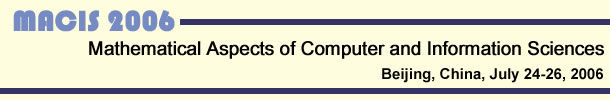Home Topics Program Submission Important Dates Invited Speakers Organization Registration Publication Poster Venue

Topics

Specific topics for MACIS 2006 include (but are not limited to):

• Modeling and Analysis of Complex Systems
Mathematical modeling and dynamics of complex systems; symbolic and numeric analysis of complex systems; stability, sensitivity, and complexity analysis; stochastic dynamic systems; physical systems; biological systems; modeling of space materials and environments; information processing in complex systems; statistical physics; computer simulation; computational techniques and tools
• Symbolic and Numeric Computation
Algebraic, symbolic, and symbolic-numeric methods for equation solving, linear and polynomial algebra, ODE/PDE, and dynamic systems; geometric computing, reasoning, and modeling; software design and implementation; applications in mathematics, statistics, system science, computer science, physical and biological sciences, engineering, economics, and finance
• Algorithms and Complexity
Algorithm design and implementation; symbolic and numeric algorithms; parallel and distributed algorithms; algebraic and geometric algorithms; analysis of algorithms; complexity theory; theoretical and practical complexity analysis; computability theory; automata theory